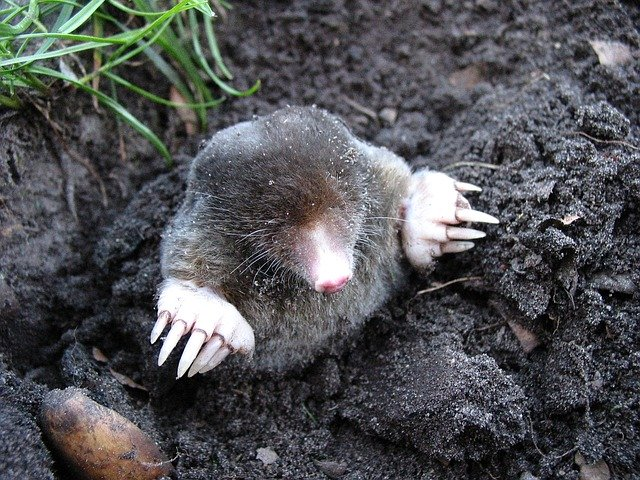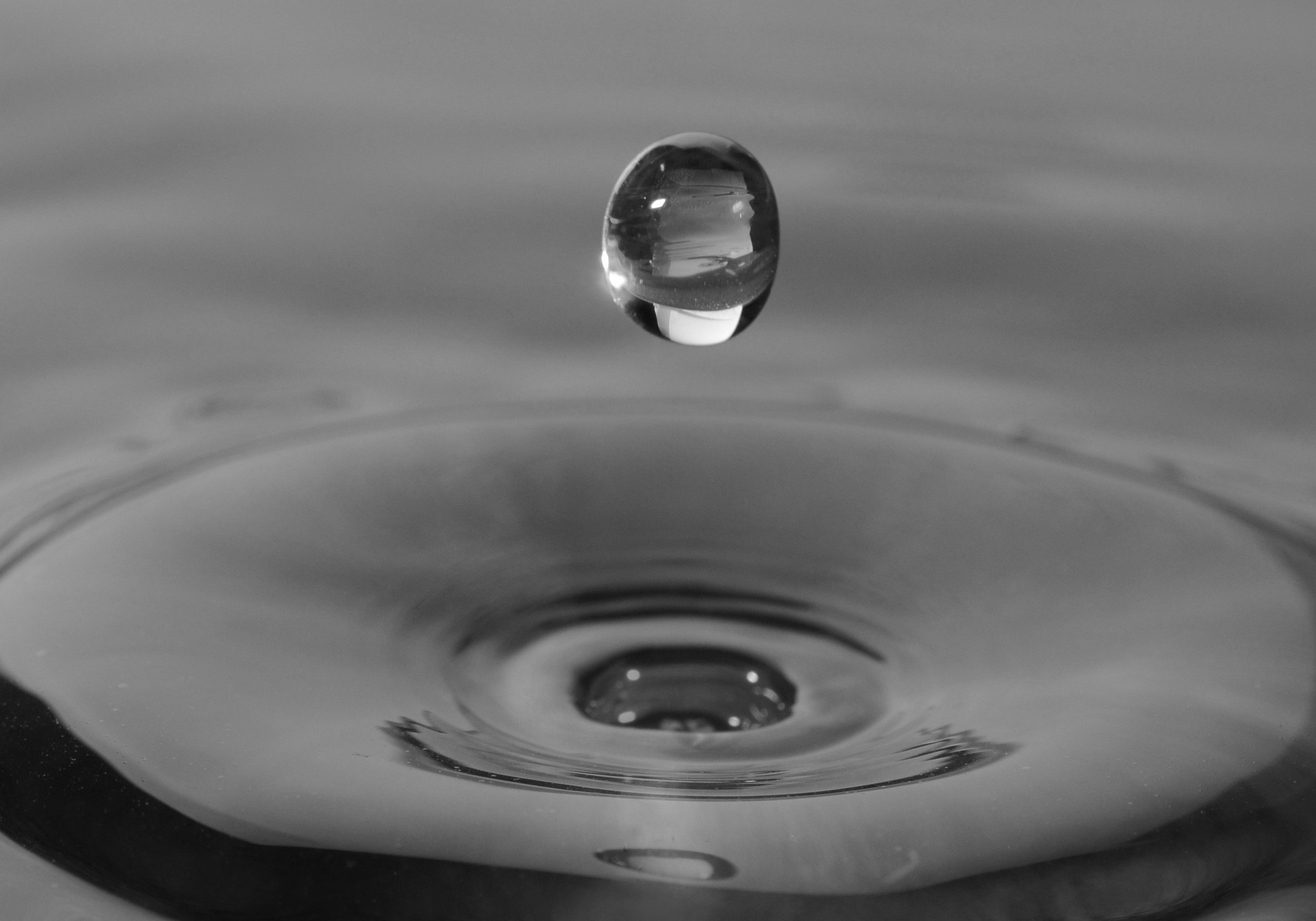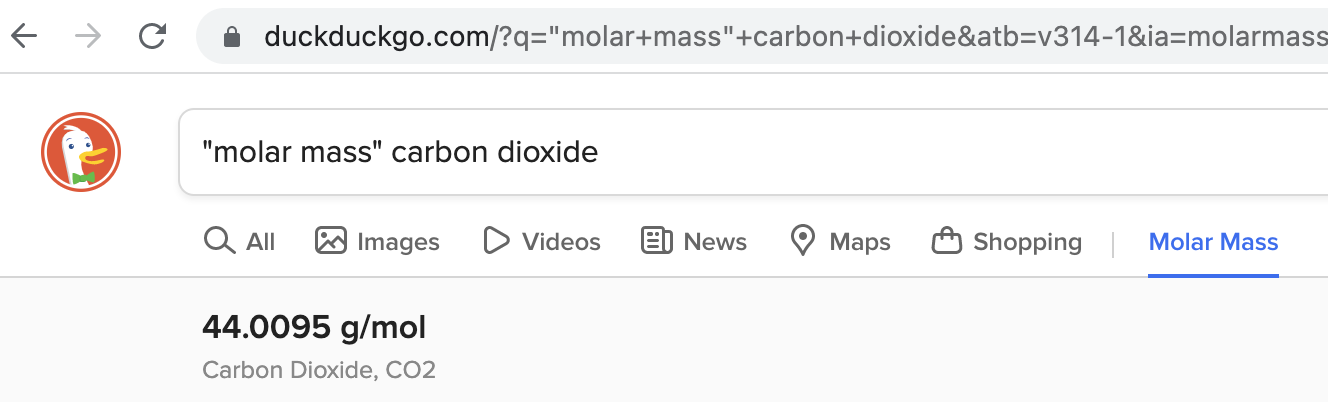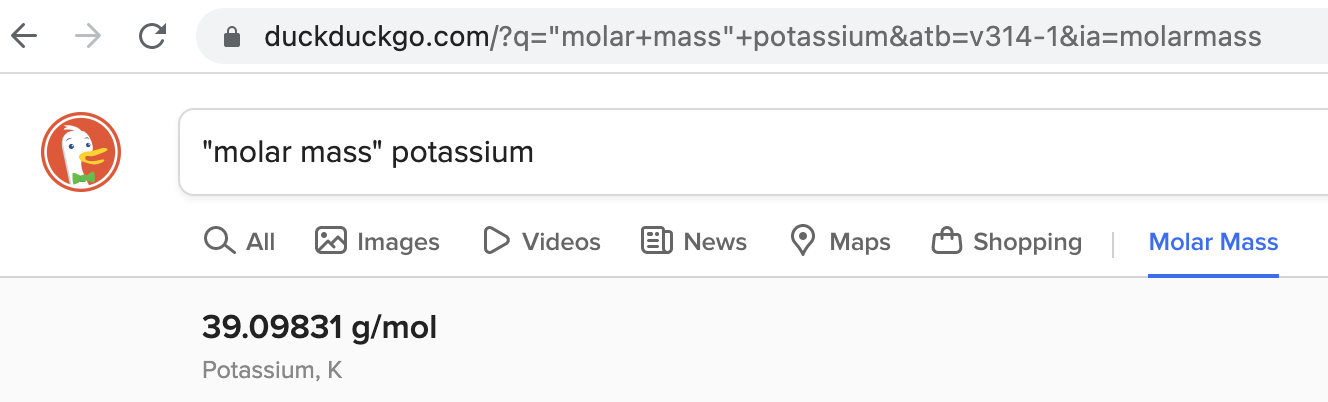12 Jun 2022 ~ 10 MIN READ

# A cup of coffee...and a mole of donutsThis post removes some of the "mystery" of moles in measures of concentration for those new to aquaculture water quality.

It includes a practical example of converting between moles and mass plus an enhanced conversion tool.

[Top]

### Plain-language Summary

Gunning Fog Index: 8.40

A mole is a grouping measure: a collection of things, like molecules or ions.

Grouping measures make large numbers smaller. Smaller numbers are easier to communicate and use in calculations.

A mole is like other grouping measures: pair (two), trio (three), dozen (twelve), and the archaic score (20).

A drop of culture water has a remarkably large number of molecules & ions.

That means that we need a very large grouping measure to work with water chemistry.

A mole is such a very large group. One mole is a group of

602,214,076,000,000,000,000,000

We need both moles and mass units to manage aquaculture water quality.

Each substance has a molar mass used to convert between mass & moles.

This post includes a software tool that simplifies converting between moles and mass.

[Top]

## A Mole Problem

We're all familiar with measures of mass (pounds, kilograms, grams...) and volume (gallons, cubic meters, liters...).

But when we first come across moles — in a Chem class or just starting with water quality — we're in unfamiliar territory.

That can be confusing.

We'll remove some of that confusion below.

[Top]

## Grouping Measures

A mole is a grouping measure.

A grouping measure is a collection of identical objects.

We already know some common grouping measures: pair (a collection of two); trio (3); quartet (4); dozen (12).

And then there's the archaic score (20), which Lincoln famously used in the opening of his Gettysburg Address:

"Four score and seven years ago..." (i.e. 4 times 20 plus 7 = 87)

#### Why do we use grouping measures?

We use them for convenience.

Grouping measures make large numbers smaller, and smaller numbers are easier to think about, communicate, & manipulate.

For example, I can't form as clear a mental image of 78 eggs as I can of the equivalent 6 1/2 dozen eggs.

#### Dozens are too small for water chemistry

Water-quality work involves unimaginably large numbers of dissolved molecules and ions.A single drop of seawater — that's only ~1/20 of a milliliter or 0.00176 ounce — has about 14.5 million trillion sodium ions.

We simply cannot relate that gargantuan number — 14.5 million trillion — to anything in our experience; but we can get a more human-friendly number with a grouping measure.

Using dozens, however, doesn't help:

To write 14.5 million trillion as dozens, we divide by 12 and get ~1.2 million trillion dozen sodium ions — still humongous.

We need a massive grouping measure to wrangle massive numbers down to our size; that's where moles come in.

[Top]

## 6.02214076 x 1023...things

A dozen is a collection of 12 and a mole is a collection of

602,214,076,000,000,000,000,000

In words:

six hundred two sextillion,
two hundred fourteen quintillion,

You often will see it written compactly in scientific notation:

6.02214076 x 1023

or with only its first two decimal places:

6.02 x 1023

23 October, 6:02 AM – 6:02 PM (6:02 10/23 in some time-date formats), is Mole Day.

#### How big is really big?

A mole is too big of a quantity to grasp. Intro Chem classes approach this in fanciful ways.• a mole of donuts would cover Earth to a depth of ~8 km (5 miles)
• a human contains an average of ~3.72 x 1013 cells (E. Bianconi et al., 2013). With ~7.8 billion people on Earth, that's "only" about 1/2 mole of human cells
• if one mole of dollar bills were divided equally among each of those 7.8 billion people, and if a person counted their share of bills at the rate of one per second, it would take almost 2.5 million years to complete the task

Just too big to grasp.

The mole is a fundamental unit...

The mole (mol) is one of the seven fundamental units in the International System of Units (Système international d'unités or SI).

It had been defined as the number of atoms in 0.012 kg of carbon-12, known as Avogadro's Number after the early 19th century Italian scientist Amedeo Avogadro.

But the kilogram was re-defined and so was the number of items in a mole.

So, on 20 May 2019, one mole was re-defined as the amount of a substance with exactly 6.02214076 × 1023 "things" -- compounds, molecules, ions, or donuts...

#### Moles of Na+ in a drop of water

We saw that a drop of seawater has 14.5 million trillion Na+ ions.

Dividing by 12, we got 1.2 million trillion dozen Na+ ions/drop.

To get that in moles, instead of dividing by 12 we divide by

6.02214076 x 1023

And let's express that in liters, the volume we commonly use in aquaculture. Without showing the math, that gives us

0.48 mol Na+ / L

That's almost one-half of a mole of Na+ ions in a liter of seawater — a number that is much easier to understand and communicate.

It's still a massive number of Na+ ions; we just made it easier to grasp by wrapping it with a grouping measure.

## We need both moles and mass

We need mass units to measure chemicals for water-quality adjustments and analyses.

Most aquaculturists also are more comfortable reporting and discussing water quality in terms of mass, not moles.

So...why do we need moles?

We need them because chemical substances — molecules & ions — combine according to their numbers, not their mass.

Those numbers are positive integers: 1, 2, 3, 4...

e.g., one sodium carbonate (Na2CO3) molecule dissolved in water produces two sodium ions (Na+) and one carbonate ion (CO3=):

one Na2CO3two Na+ + one CO3=

It's impractical to deal with one Na2CO3 molecule when we're working with the massive amounts of dissolved substances in a liter of culture water, so we interpret those numbers as moles:

one mole of Na2CO3two moles of Na+ + one mole of CO3=

[Optional] Both sides of a reaction must balance...

For each atom appearing in a reaction, the number on the left side must be the same as the number on the right side.

Above, the two sodium atoms (Na) in the Na2CO3 molecule on the left balance the two sodium ions (Na+) on the right.

It's the same for electical charge: Both sides must have the same net charge.

So, if one side is neutral, then the other side must be neutral; if one side has a minus-two (-2) net charge, then the other side must have a minus-two net charge.

Note that both sides are neutral in the sodium carbonate example: On the right-hand side the two negative charges carried by the single CO3= are cancelled by the two positive charges of the pair of Na+ ions.

Such reactions — expressed in moles — are the source of the quantitative procedures we use to manage culture water.

But we must convert that information to mass units when we measure the chemicals needed for our water-quality work.

Another time to convert between moles and mass: When academic papers report results in moles but we prefer mass.

## Conversions: Molar mass

Conversions can be tedious, but going between moles and mass isn't so complicated.

It hinges on one number — the molar mass.

Aptly named, the molar mass is simply the mass of one mole of a substance, usually in grams/mole (g/mol).

You'll also see it as milligrams/millimole (mg/mmol)
and micrograms/micromole (µg/µmol). Note that:

1 g/mol is the same as 1 mg/mmol is the same as 1 µg/µmol
1 gram per mole = 1 milligram per millimole = 1 microgram per micromole

FYI: "molar mass" is not the same as "molecular weight"

You'll often see those terms used interchangeably, but they're different:

• molar mass – mass of one mole of a substance (g/mol or mg/mmol)

• molecular weight – mass of a molecule of a substance (atomic mass units, amu)

(Note: We're not insisting on the distinction between mass and weight here.)

Each substance has a characteristic molar mass. You can find it with a simple online search as in the image below.### An example using molar mass

Let's do a practical example of the sort you might come across in the aquaculture literature. This is from a study of a sub-tropical shrimp pond (Zhang, Y. et al., 2019):

"The average...CO2 concentration...in the shrimp ponds...varied from 22.79...to...186.66 μmol L-1..."

Note 1: A micro-mole (µmol) is one-millionth of a mole; i.e., 1 mol has 1,000,000 µmol.
Note 2: The negative exponent in L-1 might be unfamiliar. When you see a negative exponent, think: fraction. So L-1 is the same as 1/L, and 186.66 μmol L-1 = 186.66 μmol / L.

You want to compare Zhang et al.'s maximum, 186.66 µmol/L, with your current CO2: 12 mg/L.

You need the molar mass of CO2 given above: ~44 g/mol.

You also know that 44 g/mol is the same as 44 µg/µmol.

1. multiply their 186.66 µmol/L by the molar mass, cancelling µmol
(186.66 µmol / L) (44 µg / µmol) = 8,215 µg CO2/L
1. one mg has 1,000 µg, so divide by 1,000 to change µg to mg, cancelling µg
(8,215 µg / L) (1 mg / 1000 µg) = 8.215 mg CO2/L

Now we've got our preferred units, and we see that your 12 mg/L is 46% higher than their 8.2 mg/L.

[Top]